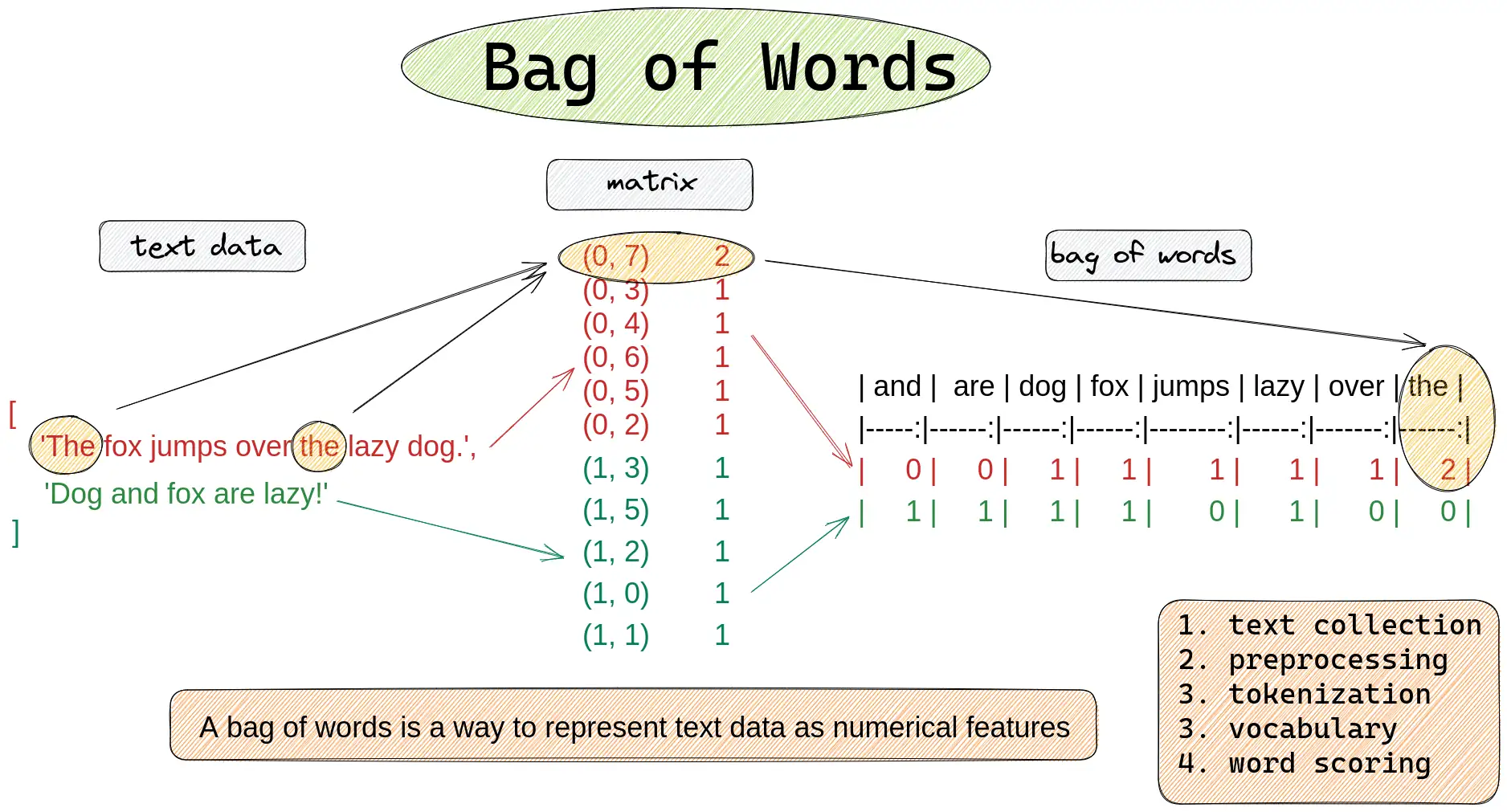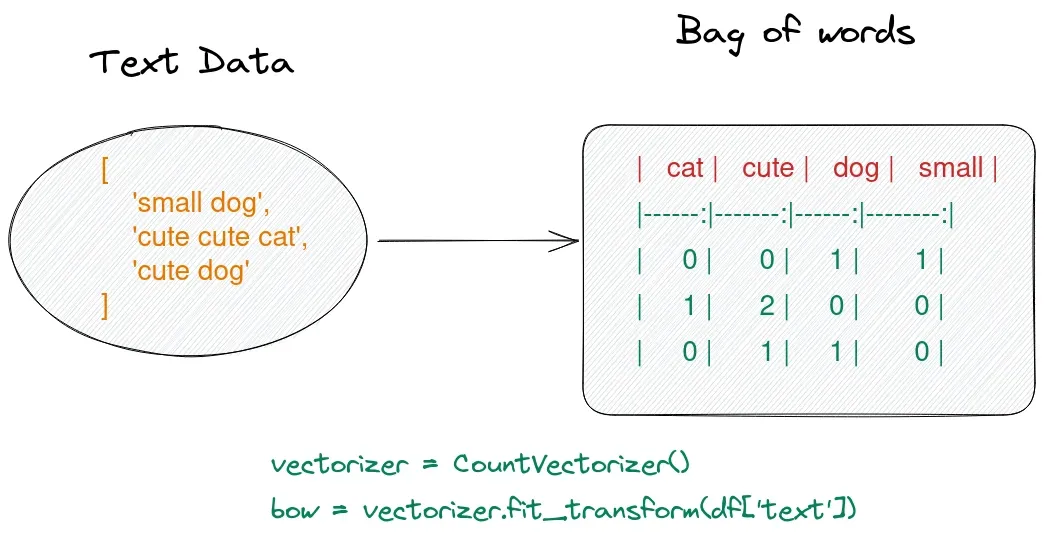In this short guide, I'll show you how to create a bag of words with Pandas and Python. You can find a example of bag of words using the `sklearn` library:

``````from sklearn.feature_extraction.text import CountVectorizer
import pandas as pd

text = ['The fox jumps over the lazy dog.',  'Dog and fox are lazy!']
data = {'text': text}
df = pd.DataFrame(data)

vectorizer = CountVectorizer()
bow = vectorizer.fit_transform(df['text'])
print(bow)

count_array = bow.toarray()
features = vectorizer.get_feature_names()
df = pd.DataFrame(data=count_array, columns=features)
``````

Below you can find the result of the code:In the next steps I'll explain the process in more detail.

## What is a Bag of Words?

A bag of words is a way to represent text data in tabular form as numerical features.

You can also find a quick solution only with Pandas and Python below:

``````import pandas as pd
from collections import Counter

text = ['Periods of rain', 'Mostly cloudy, a little rain', 'Mostly cloudy', 'Intervals of clouds and sun', 'Sunshine and mild']

df = pd.DataFrame({'text': text})
pd.DataFrame(df['text'].str.split().apply(Counter).to_list())
``````

The input DataFrame is:

text
0 Periods of rain
1 Mostly cloudy, a little rain
2 Mostly cloudy
3 Intervals of clouds and sun
4 Sunshine and mild

The output bag of words represented again as DataFrame:

Periods of rain Mostly cloudy, a little cloudy Intervals clouds and sun Sunshine mild
0 1.0 1.0 1.0 NaN NaN NaN NaN NaN NaN NaN NaN NaN NaN NaN
1 NaN NaN 1.0 1.0 1.0 1.0 1.0 NaN NaN NaN NaN NaN NaN NaN
2 NaN NaN NaN 1.0 NaN NaN NaN 1.0 NaN NaN NaN NaN NaN NaN
3 NaN 1.0 NaN NaN NaN NaN NaN NaN 1.0 1.0 1.0 1.0 NaN NaN
4 NaN NaN NaN NaN NaN NaN NaN NaN NaN NaN 1.0 NaN 1.0 1.0
Note:

For a bag of words data preprocessing is needed. Remove special characters and stopwords, convert words to lowercase, stemming etc.

The diagram below shows how to create a bag of words from multiple documents. Each item in the list is considered as separate document.## Setup

First lets create a sample DataFrame for this example:

``````from sklearn.feature_extraction.text import CountVectorizer
import pandas as pd

text = ['Periods of rain', 'Mostly cloudy, a little rain', 'Mostly cloudy', 'Intervals of clouds and sun', 'Sunshine and mild']
data = {'text': text}
df = pd.DataFrame(data)
``````

result:

text
0 Periods of rain
1 Mostly cloudy, a little rain
2 Mostly cloudy
3 Intervals of clouds and sun
4 Sunshine and mild

We are going to import `CountVectorizer` from `sklearn`.

## Step 1: Initialize the vectorizer

Next we are going to initialize the vectorizer of `sklearn`:

``````vectorizer = CountVectorizer()
``````

### lowercase

At this step we can do customization like on/off of `lowercase` conversion:

``````vectorizer = CountVectorizer(lowercase=False)
``````

### Stop words

or adding custom stop words for the bag of words:

``````vectorizer = CountVectorizer(stop_words= ['on', 'off'])
``````

even using stop words based on languages:

``````coun_vect = CountVectorizer(stop_words='english')
``````

### max_df / min_df

The abbreviation `df` in `max_df` / `min_df` stands for `document frequency`.

• `max_df = 0.75` - ignore terms that appear in more than 75% of the documents.
• `max_df = 0.5` - ignore terms that appear in less than 50% documents.

When using a float in the range `[0.0, 1.0]` they refer to the document frequency.

They can be used also in sense of `max_df = 10` - which means ignore terms that appear in less than 10 documents

``````coun_vect = CountVectorizer(max_df=1)
``````

## Step 2: Fit and transform the text data

Next step is to fit and transform the text data to create a bag of words:

``````bow = vectorizer.fit_transform(df['text'])
``````

This creates a bag of words from the DataFrame column like:

``````(0, 8)    1
(0, 7)    1
(0, 9)    1
(1, 9)    1
(1, 6)    1
(1, 2)    1
(1, 4)    1
(2, 6)    1
(2, 2)    1
(3, 7)    1
(3, 3)    1
(3, 1)    1
``````

This is a sparse matrix, where:

• each row represents a document
• each column represents a word
• the values in the matrix represent the number of times that word appears in that document.

So in `(0, 8) 1` we have:

• 0 is the number of the document
• first document
• 8 number of the feature
• periods
• 1 - is the count
• 1 occurrence

## Step 3: Get features names and counts

We can get the word list from the vectorizer, by calling the method `get_feature_names()`:

``````# get count array and features
count_array = bow.toarray()
features = vectorizer.get_feature_names()

# create DataFrame as bag of words
df = pd.DataFrame(data=count_array, columns=features)
``````

This will create a DataFrame where:

• each column is a word
• row represents the documents
• values are number of times each word is present
and clouds cloudy intervals little mild mostly of periods rain sun sunshine
0 0 0 0 0 0 0 1 1 1 0 0
0 0 1 0 1 0 1 0 0 1 0 0
0 0 1 0 0 0 1 0 0 0 0 0
1 1 0 1 0 0 0 1 0 0 1 0
1 0 0 0 0 1 0 0 0 0 0 1

## Normalize the bag of words

Alternatively, we can also use `TfidfVectorizer` from `scikit-learn` that creates a bag of words by:

• first counting the frequency of each word in each document
• then normalizing the resulting counts by dividing by the total number of words in the document.

The resulting values are the term frequency-inverse document frequency (TF-IDF) values:

``````from sklearn.feature_extraction.text import TfidfVectorizer
import pandas as pd

text = ['small dog', 'cute cat', 'cute dog', 'cat']
data = {'Text':text}
df = pd.DataFrame(data)

vectorizer = TfidfVectorizer()
bow = vectorizer.fit_transform(df['Text'])
print(bow)
``````

result:

``````(0, 2)    0.6191302964899972
(0, 3)    0.7852882757103967
(1, 0)    0.7071067811865475
(1, 1)    0.7071067811865475
(2, 1)    0.7071067811865475
(2, 2)    0.7071067811865475
(3, 0)    1.0
``````

Getting bag of words as a DataFrame with normalized values:

``````count_array = bow.toarray()
features = vectorizer.get_feature_names()
df = pd.DataFrame(data=count_array, columns=features)
``````
cat cute dog small
0.000000 0.000000 0.619130 0.785288
0.707107 0.707107 0.000000 0.000000
0.000000 0.707107 0.707107 0.000000
1.000000 0.000000 0.000000 0.000000

## Conclusion

To summarize, in this article, we've seen examples of bags of words. We've briefly covered what a bag of words is and how to create it with Python, Pandas and scikit-learn.

And finally, we've seen how to normalize a bag of words with scikit-learn.